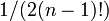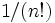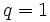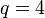# Group cohomology of finite homocyclic groups

Jump to: navigation, search
This article gives specific information, namely, group cohomology, about a family of groups, namely: homocyclic group.
View group cohomology of group families | View other specific information about homocyclic group

We are interested in describing the homology groups and cohomology groups for a homocyclic group of the form$(\mathbb{Z}/m\mathbb{Z})^n$. This can be viewed as the additive group of a free$m$-dimensional module over the ring$\mathbb{Z}/m\mathbb{Z}$. It is isomorphic to the external direct product of$n$ copies of the finite cyclic group of order$m$.

## Related discussions

For this article, we will use the following notation:

•$m$ is the exponent of the group, i.e., the order of the underlying cyclic group.
•$n$ is the minimum size of generating set for the group, i.e., its rank as a free module over the ring$\mathbb{Z}/m\mathbb{Z}$.
•$q$ is the degree in which we are looking at the homology or cohomology, i.e., we are looking at$H_q$ or$H^q$.

## Homology groups

FACTS TO CHECK AGAINST (homology group for trivial group action):
First homology group: first homology group for trivial group action equals tensor product with abelianization
Second homology group: formula for second homology group for trivial group action in terms of Schur multiplier and abelianization|Hopf's formula for Schur multiplier
General: universal coefficients theorem for group homology|homology group for trivial group action commutes with direct product in second coordinate|Kunneth formula for group homology

### Over the integers

All the formulas obtained here are obtained by combining information about the group cohomology of finite cyclic groups with the Kunneth formula for group homology, as well as basic facts about computation of tensor products and$\operatorname{Ext}$ for finitely generated abelian groups. Details of the derivations are pending.

#### Rank as polynomial in homology degree for fixed rank of homocyclic group

The zeroth homology group is always$\mathbb{Z}$. All higher homology groups are themselves homocyclic groups with exponent$m$, i.e., they are free modules over the ring$\mathbb{Z}/m\mathbb{Z}$. For fixed$n$, there are two polynomials in$q$, both of degree$n - 1$ (one for even$q$, one for odd$q$) such that the rank of$H_q((\mathbb{Z}/m\mathbb{Z})^n;\mathbb{Z})$ is that polynomial in$q$. The polynomials are given below. In all cases, the following are true:

• Both polynomials have degree$n - 1$.
• For$n > 1$, the leading coefficient of both polynomials is$1/(2(n - 1)!)$ (is it? Just guesswork right now).
• The polynomials differ only in their constant terms, with the polynomial for even$q$ having zero constant term.
Value of$n$ Polynomial that gives rank of$H_q((\mathbb{Z}/m\mathbb{Z})^n;\mathbb{Z})$ for odd positive$n$ Polynomial that gives rank of$H_q((\mathbb{Z}/m\mathbb{Z})^n;\mathbb{Z})$ for even positive$n$ Degree of the polynomials (equals$n - 1$) Average of leading coefficients for even and odd (equals$1/(2(n - 1)!)$; note that both leading coefficients are equal to this for$n > 1$) Constant term for polynomial for odd degree, which equals that polynomial minus the polynomial for even degree (equals$2 - (1/2^{n-1})$) Description of polynomial for odd$q$ in terms of$u = (q + 1)/2$ Description of polynomial for even$q$ in terms of binomial polynomials of$u = q/2$
1 1 0 0 1/2 1 1 0
2$\frac{q + 3}{2}$$\frac{q}{2}$ 1 1/2 3/2$\binom{u}{0} + \binom{u}{1}$$\binom{u}{1}$
3$\frac{q^2 + 4q + 7}{4}$$\frac{q^2 + 4q}{4}$ 2 1/4 7/4$\binom{u}{0} + 2\binom{u}{1} + 2\binom{u}{2}$$3\binom{u}{1} + 2\binom{u}{2}$
4$\frac{2q^3 + 15q^2 + 34q + 45}{24}$$\frac{2q^3 + 15q^2 + 34q}{24}$ 3 1/12 15/8$\binom{u}{0} + 3\binom{u}{1} + 7\binom{u}{2} + 4\binom{u}{3}$$6\binom{u}{1} + 9\binom{u}{2} + 4\binom{u}{3}$

#### Rank as polynomial in rank for fixed degree of homocyclic group

The zeroth homology group is always$\mathbb{Z}$. All higher homology groups are themselves homocyclic groups with exponent$m$, i.e., they are free modules over the ring$\mathbb{Z}/m\mathbb{Z}$. For fixed$q$, we can find a polynomial in$n$ such that the rank of$H_q((\mathbb{Z}/m\mathbb{Z})^n;\mathbb{Z})$ is that polynomial in$q$. The polynomials are given below:

Value of$q$ Polynomial in$n$ that gives rank of$H_q((\mathbb{Z}/m\mathbb{Z})^n;\mathbb{Z})$ Degree (equals$n$) Leading coefficient (equals$1/(n!)$) Description in terms of binomial polynomials
1$n$ 1 1$\binom{n}{1}$
2$\frac{n(n - 1)}{2}$ 2 1/2$\binom{n}{2}$
3$\frac{n(n^2 + 5)}{6}$ 3 1/6$\binom{n}{1} + \binom{n}{2} + \binom{n}{3}$

#### Combined information on ranks

Below are the ranks of the homology groups$H_q((\mathbb{Z}/m\mathbb{Z})^n;\mathbb{Z})$ in terms of both$n$ and$q$.$n$ (rows),$q$ (columns) Polynomial for odd$q$ for fixed$n$ Polynomial for even$q$ for fixed$n$ Rank for$q = 1$ Rank for$q = 2$ Rank for$q = 3$ Rank for$q = 4$ Rank for$q = 5$
Polynomial in$n$ for fixed$q$ -- --$n$$\frac{n(n-1)}{2}$$\frac{n(n^2 + 5)}{6}$  ?  ?
Case$n = 1$ 1 0 1 0 1 0 1
Case$n = 2$$\frac{q + 3}{2}$$\frac{q}{2}$ 2 1 3 2 4
Case$n = 3$$\frac{q^2 + 4q + 7}{4}$$\frac{q^2 + 4q}{4}$ 3 3 7 8 13
Case$n = 4$$\frac{2q^3 + 15q^2 + 34q + 45}{24}$$\frac{2q^3 + 15q^2 + 34q}{24}$ 4 6 14 21 35